# Search

About 13 Search Results Matching Types of Worksheet, Worksheet Section, Generator, Generator Section, Subjects matching Greater Than\, Less Than Practice, Similar to Reading a Protractor Worksheet 2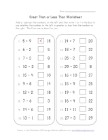## Greater and Less Than Equations Worksheet

Practice greater than, less than and equals wit...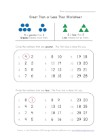## Less and Greater Worksheet Two

One section asks kids to find the numbers that ...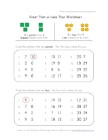## Less and Greater Worksheet One

Follow the instructions to find all the numbers...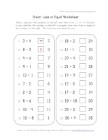## Greater, Less and Equal Worksheet

Perform the basic math equations and then fill ...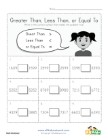## Greater Than, Less Than or Equal To - Thousands

Look at the two numbers in each section and dec...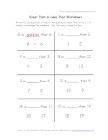## Greater Than Less Than

Write the word "greater" or "less" and circle t...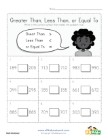## Greater Than, Less Than or Equal To - 100 to 1000

Look at the two numbers in each section and dec...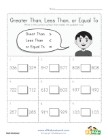## Greater Than, Less Than or Equal To - Hundreds

Look at the two numbers in each section and dec...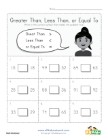## Greater Than, Less Than or Equal To - Numbers 1 through 100

Look at the two numbers in each section and dec...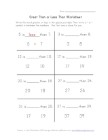## Greater and Less

Complete the sentence by writing “greater” or “...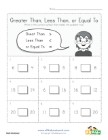## Greater Than, Less Than or Equal To - Numbers 1 through 20

Look at the two numbers in each section and dec...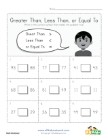## Greater Than, Less Than or Equal To - 1 to 100

Look at the two numbers in each section and dec...